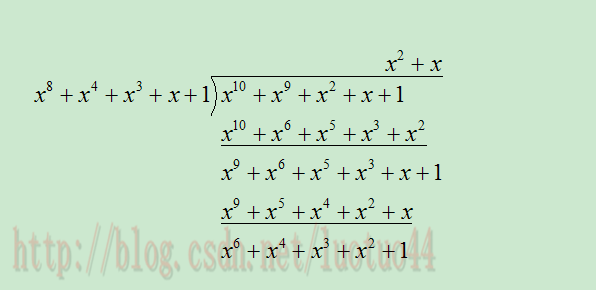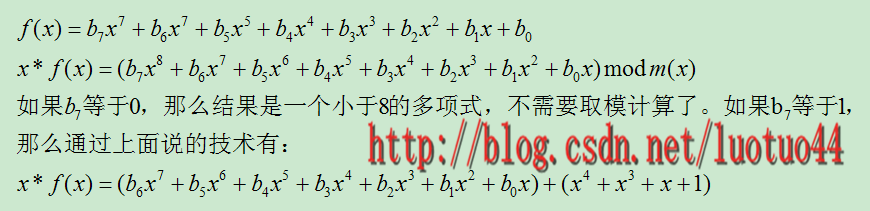# 域的性质：

在数学上的概念就不解释，可以参考维基百科。当然也可以参考《密码编码学与网络安全》这书的有限域一章。形象地说，域有这样一个性质：在加法和乘法上具有封闭性。也就是说对域中的元素进行加法或乘法运算后的结果仍然是域中的元素。有一点要注意，域里面的乘法和加法不一定是我们平常使用的乘法和加法。可以把C语言中的与运算和异或运算分别定义成加法和乘法。但习惯上，仍然使用符号+ 和 * 表示加法和乘法运算。

本文会简单介绍一些有关群和域的概念，不过对于概念的定义，本文写得并不严谨，所以对于这些概念，最好还是配合书或者维基百科一起看吧。

域有单位元和逆元两个概念。

加法和乘法运算都有对应的单位元(这两个单位元一般不同，但都用符号e表示)。单位元就像线性代数的单位矩阵。一个矩阵乘以单位矩阵等于本身。对应地，在域中的单位元有：对于加法单位元，所有元素加上单位元e，等于其本身。对应乘法单位元，所有元素乘上单位e，等于其本身。

逆元就像数学上的倒数，两个元素互为对方的逆元。如果元素a和b互为加法逆元，那么就有 a + b = e。若互为乘法逆元，那么就有a * b = e。如果元素a在域中找不到另外一个元素b，使得a+b=e(a*b=e)，那么a就没有加法(乘法)逆元。

逆元有什么用呢？其实逆元是用于除法运算的。小学的时候老师都会教：除于一个分数就等于乘以该分数的倒数(分数的倒数就是该分数的乘法逆元)。所以要想除于某个数，可以乘以该数的逆元。

一个集合有加法单位元和乘法单位元，以及每一个元素都对应有加法逆元和乘法逆元，是成为域的必要条件。需要注意：即使集合里面有元素0，并且0没有对应的乘法逆元，那么该集合也可能是一个域。因为并不要求0有乘法逆元。

一个域的例子就是我们平时熟悉的有理数集合，相应的加法和乘法就是我们平时用的加法和乘法。其中，加法的单位元为0，有理数a的加法逆元就是其相反数。因为a + (-a) = 0(单位元)。乘法的单位元为1，a的乘法逆元是其倒数。因为a * (1/a) = 1。注意这里的元素0并没有乘法逆元。

# 有限域：

有限域，是指域中的元素个数是有限的。

## 有限域GF(p)：

在密码学中，有限域GF(p)是一个很重要的域，其中p为素数。简单来说，GF(p)就是 mod p，因为一个数 模p后，结果在[0, p-1]之间。对于元素a和b，那么(a+b) mod p和(a*b)mod p，其结果都是域中的元素。GF(p)里面的加法和乘法都是平时用的加法和乘法。GF(p)的加法和乘法单位元分别是0和1，元素的加法和乘法逆元都很容易理解和求得，这里就不展开讲了，《密码编码学与网络安全》书中有详讲的。求乘法逆元的实现代码如下面所示，具体是使用了类似辗转相除法的方法。

//g_prime就是 GF(p)中的p
int g_prime = 251;

int calculateInverse(int x)
{
int a1 = 1, a2 = 0, a3 = g_prime;
int b1 = 0, b2 = 1, b3 = x;

while( 1 )
{
if( b3 == 0 )
throw std::logic_error("should not be 0");

if( b3 == 1 )
break;

int q = a3 / b3;

int t1 = a1 - q*b1, t2 = a2 - q*b2, t3 = a3 - q*b3;

a1 = b1; a2 = b2; a3 = b3;
b1 = t1; b2 = t2; b3 = t3;
}

return (b2 + g_prime)%g_prime;
}


有一个问题，读者可能会疑惑，为什么p一定要是一个素数呢？这是因为当p为素数时，才能保证集合中的所有的元素都有加法和乘法逆元(0除外)。

假如p等于10，其加法和乘法单位元分别是0和1。加法没有问题，所有元素都有加法逆元，但对于乘法来说，比如元素2，它就没有乘法逆元。因为找不到一个数a，使得2*a mod 10等于1。这时，就不能进行除于2运算了。

对于p等于素数，那么它就能保证域中的所有元素都有逆元。即，对于域中的任一个元素a，总能在域中找到另外一个元素b，使得a*b mod p 等于1。这个是可以证明的，利用反证法和余数的定义即可证明，不难。

## 有限域GF(2^8)：

现在重点讲一下GF(2^n)，特别是GF(2^8)，因为8刚好是一个字节的比特数。

前面说到， GF(p)，p得是一个素数，才能保证集合中的所有元素都有加法和乘法逆元(0除外)。但我们却很希望0到255这256个数字也能组成一个域。因为很多领域需要用到。mod 256的余数范围就是0到255，但256不是素数。小于256的最大素数为251，所以很多人就直接把大于等于251的数截断为250。在图像处理中，经常会这样做。但如果要求图像无损的话，就不能截断。

貌似已经到了死胡同，救星还是有的，那就是GF(p^n)，其中p为素数。在这里我们只需令p为2，n为8，即GF(2^8)。

## 多项式运算：

要弄懂GF(2^n)，要先明白多项式运算。这里的多项式和初中学的多项式运算有一些区别。虽然它们的表示形式都是这样的:f(x) = x^6 + x^ 4 + x^2 + x + 1。下面是它的一些特点。

1. 多项式的系数只能是0或者1。当然对于GF(p^n)，如果p等于3，那么系数是可以取：0， 1， 2的
2. 合并同类项时，系数们进行异或操作，不是平常的加法操作。比如x^4 + x^4等于0*x^4。因为两个系数都为1， 进行异或后等于0
3. 无所谓的减法(减法就等于加法)，或者负系数。所以，x^4 – x^4就等于x^4 + x^4。-x^3就是x^3。

看一些例子吧。对于f(x) = x^6 + x^4 + x^2 + x + 1。g(x) = x^7 + x + 1。

那么f(x) + g(x)  = x^7 + x^6 + x^4+ x^2 + (1+1)x + (1+1)1 = x^7 + x^6 + x^4 + x^2。f(x) – g(x)等于f(x) + g(x)。

f(x) * g(x) =(x^13 + x^11 + x^9 + x^8 + x^7)  +  (x^7 + x^5 + x^3 + x^2 + x)  +  (x^6+ x^4 + x^2 + x + 1) = x^13 + x^11 + x^9 + x^8 + x^6 + x^5+ x^4+ x^3+1。

下图是除法，除法得到的余数，也就是mod操作的结果。## 素多项式：

对于多项式也类似素数，有素多项式。其定义和素数类似，素多项式不能表示为其他两个多项式相乘的乘积。

## 素多项式模运算：

指数小于3的多项式有8个，分别是0， 1， x， x+1， x^2， x^2+1， x^2 + x， x^2+x+1。对于GF(2^3)来说，其中一个素多项式为x^3+x+1。上面8个多项式进行四则运算后 mod (x^3+x+1)的结果都是8个之中的某一个。当然也可以证明这是一个域，所以每一个多项式都是有加法和乘法逆元的(0除外)。注意，这些逆元都是和素多项式相关的，同一个多项式，取不同的素多项式，就有不同的逆元多项式。

对于GF(2^8)，其中一个素多项式为x^8 + x^4 + x^3 +x +1。对应地，小于8次的多项式有256个。

由素多项式得到的域，其加法单位元都是0，乘法单位元是1

重点来了：

前面讲到了对素多项式取模，然后可以得到一个域。但这和最初的目的有什么关系吗？多项式和0， 1， ……，255没有什么关系。确实是没有什么关系，但多项式的系数确可以组成0， 1， 2，……255这些数。回到刚才的GF(2^3),对应的8个多项式，其系数刚好就是000,001, 010, 011, 100, 101, 110, 111。这不正是0到7这8个数的二进制形式吗？也就是说，它们有一一对应映射的关系。多项式对应一个值，我们可以称这个值为多项式值

对于GF(2^3)，取素多项式为x^3 + x+1，那么多项式x^2+x的乘法逆元就是x+1。系数对应的二进制分别为110和011。此时，我们就认为对应的十进制数6和3互为逆元。即使mod 8不能构成一个域，但通过上面的对应映射，0到7这8个数一样有对应逆元了(为了顺口，说成0到7。实际0是没有乘法逆元的)。同样，对于GF(2^8)也是一样的。所以0到255，这256个数都可以通过这样的方式得到乘法逆元(同样，0是没有乘法逆元的)。

## GF(2^8)的四则运算：

其实，通过前面的讲解，已经可以对GF(2^8)进行四则运算了。但计算起来相当麻烦。接下来就是讲解一下怎么用简单的方法进行四则运算，以及编程的实现(对于码农来说，这才是终极目标啊)。

下面讲解的所有运算，默认的素多项式为x^8 +x^4 + x^3 +x +1,m(x)表示。GF(2^8)的素多项式有多个，但这个经典啊。

### 加法和减法：

加法和减法就是经典的异或运算，没有什么可说的。

### 乘法：

前面的一个多项式相乘例子有说到怎么进行相乘计算，但过于复杂。《密码编码学与网络安全》一书说到了一个计算乘法的技巧。

首先有，x^8 mod m(x) = [m(x) – x^8] = x^4 + x^3 +x +1。

对于多项式f(x)， 有：对于C语言来说，通过位移运算符<< 和 异或运算，很容易计算。对于x的指数高于一次的情况，可以通过递归的形式使用。如：x^2 * f(x) = x*[x*f(x)]。

虽然有上面的技巧，但还是过于复杂。在大量运算中(比如图像处理)，耗时太多。于是人们就想到了通过查表的形式计算。

要弄懂查表的原理，得明白一个概念：生成元g

首先，在群中定义幂运算为重复运用群的运算符。假如运算符为普通的加法，那么幂运算就是多个加法一起使用。

如果元素g满足下面的条件，我们就称g为生成元：对于集合中的任何的一个元素，都可以通过元素g的幂g^k得到。并定义g^0 = e，假设h为g的逆元，那么还定义g^(-k) = h^k。比如，整数集合，都可以由生成元1得到。2 = 1 + 1 = 1^2、3 = 1^3=1 + 1 + 1、……。负数可以通过幂取负数得到。

将生成元应用到多项式中， GF(2^n)中的所有多项式都是可以通过多项式生成元g通过幂求得。即域中的任意元素a，都存在一个k，使得a = g^k。

下面看一下，怎么将生成元应用到多项式乘法中。

对于g^k = a，有正过程和逆过程。知道k求a是正过程，知道了a反过来求k是逆过程。同样，假设有g^n = a和g^m = b。现在需要求a*b，那么就有a*b = g^n* g^m = g^(n+m)。我们只需要：根据a和b，分别求得n和m。然后直接计算g^(n+m)即可。求，并不是真的傻乎乎地通过计算而得到，而是通过查表。这里，构造两个表，正表和反表。正表是知道了指数，求值。反表是知道了值，求指数。接下来要做的就是构造这两个表。为了做除法运算，还要构造逆元表。

在给出三个表的构造代码前，有几个东西要讲一下。

虽然生成元g的幂次厉害，但多项式0，是无法用生成元生成的。g^0等于多项式1，而不是0。为什么？逆向思考一下：假如存在k使得g^k = 0，那么g^(k+1)等于多少呢？

GF(2^n)是一个有限域，就是元素个数是有限的，但指数k是可以无穷的。所以必然存在循环。这个循环的周期是2^n-1，因为多项式0，g不能生成，少了一个。所以对于GF（2^8），当k大于等于255时，g^k =g^(k%255)。所以对于正表，生成元的指数，取0254即可，对应地生成255个不同的多项式，多项式的取值范围为1255

有了上面的讨论，对于正表，只需依次计算g^0、g^1、g^2，……，g^254即可。对于GF(2^8)，素多项式m(x) = x^8 + x^4 + x^3 +x +1，对应的生成元g(x) = x + 1。下面是具体的实现代码：

int table;
int i;

table = 1;//g^0
for(i = 1; i < 255; ++i)//生成元为x + 1
{
//下面是m_table[i] = m_table[i-1] * (x + 1)的简写形式
table[i] = (table[i-1] << 1 ) ^ table[i-1];

//最高指数已经到了8，需要模上m(x)
if( table[i] & 0x100 )
{
table[i] ^= 0x11B;//用到了前面说到的乘法技巧
}
}


这个正表，下标值等于生成元的指数，下标对应的元素值等于对应的多项式值。

反表和正表是对应的，所以反表中元素的个数也是255个。正表中，生成元g的指数k的取值范围为0到254。多项式值g^k的取值范围为1到255。所以在反表中，下标的取值范围为1到255，元素值的取值范围为0到254。实现代码如下：

int arc_table;

for(i = 0; i < 255; ++i)
arc_table[ table[i] ] = i;


对于逆元表，先看逆元的定义。若a和b互为逆元，则有a*b = e。用生成元表示为：g^n* g^m = e = 1。又因为e = g^0 = g^255(循环，回头了)。所以g^k * g(255-k) = g^(k + 255 -k) = e。于是g^k 和 g^(255-k)互为逆元。对于多项式值val，求其逆元。可以先求val对应的g幂次是多少。即g的多少次方等于val。可以通过反向表查询， 设为k。那么其逆元的幂次为255-k。此时再通过正向表查询即可。实现代码如下：

int inverse_table;

for(i = 1; i < 256; ++i)//0没有逆元，所以从1开始
{
int k = arc_table[i];
k = 255 - k;
k %= 255;//m_table的取值范围为 [0, 254]
inverse_table[i] = table[k];
}


求完三个表后，现在总结一下三个表下标和下标对应元素值的含义。

对于正表，下标就是生成元g的指数，取值范围为[0, 254]。下标对应元素就是g^k得到的多项式值。取值范围为[1, 255]。

对于反表，下标就是g^k得到的多项式值，取值范围为[1, 255]。下标对应的元素就是生成元g的指数，取值范围为[0, 254]。

对于逆表，下标值和下标对应元素的值互为逆元，取值范围都是[1, 255]。k的逆元就是inverse_table[k]。

有了这些表，现在再去求多项式相乘，那么超级简单了。代码如下：

int mul(int x, int y)
{
if( !x || !y )
return 0;

return table[ (arc_table[x] + arc_table[y]) % 255];
}


### 除法：

除法直接使用上面的逆元表即可，所以a/b等于mul(a, inverse_table[b]);  这里b不能取0。0没有逆元，不能除于0。

# 拉格朗日插值：

拉格朗日插值是什么，可以参考维基百科。拉格朗日插值的一个很常见应用是：知道了平面上的n个点的坐标值，现在求一个函数f(x)，它经过这n个点。

在实数里面，利用拉格朗日插值法是很容易求的。但对于GF(p)和GF(p^n)，拉格朗日插值法就有点难了。一开始我甚至怀疑拉格朗日插值法能不能用于GF(p)和GF(p^n)，毕竟这两个东西的运算规则是奇葩的(特别是GF(p^n))。不得不说，拉格朗日更奇葩，他构造出来的拉格朗日插值法也能用于GF(p)和GF(p^n)。

对于GF(p)和GF(p^n)，拉格朗日插值法中的分母，直接用其逆元即可。计算起来也不是太难，用前面提到的逆元表更是容易。

## 拉格朗日插值多项式展开系数：

拉格朗日插值法中的分子就坑爹了。虽然展开后，有一些规律，但对于编程来说，是很麻烦的。

还好，在中国知网那里搜到了一篇文章，里面有讲到怎么把拉格朗日插值法中的分子展开成利于编程实现的形式。鉴于读者们可能不能在知网下载文章，所以我就把文章上传到csdn中。读者可以点这里下载，细看。这里就不讲了，直接给出实现代码。

#include <iostream>
#include<vector>

using namespace std;

int accumulate(const std::vector<int> &vec, int start, int end)
{
int i, val = 0;
while( start != end)
{
val += vec[start++];
}

return val;
}

std::vector<int> fun(std::vector<int>& vec)
{
int i, j;
int size = vec.size();

std::vector<int> factor;
std::vector<int> result(vec.size() + 1, 1);

factor.resize(size, 1);
for(j = 0; j < size; ++j)
{
std::vector<int> temp;
for(i = 0; i < size - j; ++i)
{
temp.push_back(vec[i] * factor[i]);
}

result[j + 1] = accumulate(temp, 0, temp.size());

for(i = 1; i < temp.size(); ++i)
factor[i-1] = accumulate(temp, i, temp.size());
}

return result;
}

int main()
{
int val[] = {2, 3, 5};

std::vector<int> vec(val, val + sizeof(val)/sizeof(val));

//结果数组中，依次是高最次幂的系数，次高次幂的系数....一次幂的系数，常数项的系数
std::vector<int> result = fun(vec);

int i = 0;
for(i = 0; i < result.size(); ++i)
cout<<result[i]<<' ';
cout<<endl;

return 0;
}


需要注意的是，上面代码是那篇文章的直接实现，是在实数域里面的运算。需要修改才能用于GF(2^8)。只需把代码里面的加法和乘法替换成GF(2^8)的加法和乘法即可。

05-283万+

05-011万+
10-151万+
10-22380
09-264736
06-251186
04-046202
04-043035
11-088876
10-121930
04-244054
10-121989
01-236万+
12-201654
12-081297
03-17382

### “相关推荐”对你有帮助么？

•非常没帮助
•没帮助
•一般
•有帮助
•非常有帮助luotuo44

¥2 ¥4 ¥6 ¥10 ¥20余额支付 (余额：-- )扫码支付获取中扫码支付点击重新获取扫码支付1.余额是钱包充值的虚拟货币，按照1:1的比例进行支付金额的抵扣。
2.余额无法直接购买下载，可以购买VIP、C币套餐、付费专栏及课程。余额充值